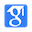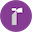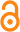# Bi-level Actor-Critic for Multi-agent CoordinationHaifeng ZhangWeizhe ChenZeren HuangMinne Linational conference on artificial intelligence, 2020.

Keywords:
level actor critic algorithmmarkov gamemodel freebi-level actorcriticdecision makingMore(9+)
Weibo:
Our experiments on matrix games and a highway merge environment demonstrate the effectiveness of our algorithm to find the Stackelberg solutions which outperform the state-of-the-art baselines

Abstract:

Coordination is one of the essential problems in multi-agent systems. Typically multi-agent reinforcement learning (MARL) methods treat agents equally and the goal is to solve the Markov game to an arbitrary Nash equilibrium (NE) when multiple equilibra exist, thus lacking a solution for NE selection. In this paper, we treat agents \emp...More

Code:

Data:

0
Introduction
• The original game model is symmetric that agents should make decision simultaneously, the authors are still able to define a priority of decision making for the agents in the training phase and keep simultaneous decision making in the execution phase
• In this asymmetric game model, the Stackelberg equilibrium (SE) (Von Stackelberg 2010) is set up as the learning objective rather than the Nash equilibrium (NE).
• The authors' empirical study shows the SE is likely to be Pareto superior to the average NE in games with high cooperation level
Highlights
• It is not able to guarantee to converge to a particular Nash equilibrium with these approaches, which leads to uncertainty and sub-optimality
• The original game model is symmetric that agents should make decision simultaneously, we are still able to define a priority of decision making for the agents in the training phase and keep simultaneous decision making in the execution phase
• In a non-cooperative case shown in Table 1b, the Stackelberg equilibrium (SE) is not included in the set of the Nash equilibrium (NE) and is Pareto superior to any NE
• We propose a novel bi-level actor-critic algorithm which is trained centrally and asymmetrically and executed decentrally and symmetrically
• Our experiments on matrix games and a highway merge environment demonstrate the effectiveness of our algorithm to find the Stackelberg solutions which outperform the state-of-the-art baselines
Results
• The authors' experiments on matrix games and a highway merge environment demonstrate the effectiveness of the algorithm to find the Stackelberg solutions which outperform the state-of-the-art baselines.
Conclusion
• The authors consider Stackelberg equilibrium as a potentially better learning objective than Nash equilibrium in coordination environments due to its certainty and optimality.
• The authors formally define the bi-level reinforcement learning problem as the multi-state model-free Stackelberg equilibrium learning problem and empirically study the relationship between the cooperation level and the superiority of Stackelberg equilibrium to Nash equilibrium.
• The authors' experiments on matrix games and a highway merge environment demonstrate the effectiveness of the algorithm to find the Stackelberg solutions which outperform the state-of-the-art baselines
Summary
• ## Introduction:

The original game model is symmetric that agents should make decision simultaneously, the authors are still able to define a priority of decision making for the agents in the training phase and keep simultaneous decision making in the execution phase
• In this asymmetric game model, the Stackelberg equilibrium (SE) (Von Stackelberg 2010) is set up as the learning objective rather than the Nash equilibrium (NE).
• The authors' empirical study shows the SE is likely to be Pareto superior to the average NE in games with high cooperation level
• ## Results:

The authors' experiments on matrix games and a highway merge environment demonstrate the effectiveness of the algorithm to find the Stackelberg solutions which outperform the state-of-the-art baselines.
• ## Conclusion:

The authors consider Stackelberg equilibrium as a potentially better learning objective than Nash equilibrium in coordination environments due to its certainty and optimality.
• The authors formally define the bi-level reinforcement learning problem as the multi-state model-free Stackelberg equilibrium learning problem and empirically study the relationship between the cooperation level and the superiority of Stackelberg equilibrium to Nash equilibrium.
• The authors' experiments on matrix games and a highway merge environment demonstrate the effectiveness of the algorithm to find the Stackelberg solutions which outperform the state-of-the-art baselines
Tables
• Table1: Coordination games. (a) A cooperative game where A-X and C-Z are the NE. C-Z is also the SE and the Perato optimality point. (b) A non-cooperative game where A-X is the SE, B-Y and C-Z are the NE. The SE is Pareto superior to any NE in this game
• Table2: Result of Escape game. The first two column means the average reward while the third column means the percentage of converging to C-Z, the global optimal point
• Table3: Result of Maintain game.The first two column means the average reward while the third column means the percentage of converging to A-X
• Table4: Result of Traffic Merge. The first column shows the rate of the car from main lane to go first. The second column shows the rate of the car from auxiliary lane to go first. And the third column shows the rate of successful merge after training
Related work
• In MARL, various approaches were proposed to tackle the coordination problem (Bu et al 2008), especially for the cooperative environments. A general approach is applying the social convention which breaks ties by ordering of agents and actions (Boutilier 1996). Our method is compatible with social convention in the sense that we find the SE as the common knowledge of the agents about the game, based on which they can form social conventions. For cooperative games, the optimistic exploration were proposed (Claus and Boutilier 1998) for reaching optimal equilibrium. Lauer and Riedmiller (2000) used maximal estimation to update Qvalue which ensures convergence to the optimal equilibrium given the reward function is deterministic. For the case of stochastic reward function, FMQ (Kapetanakis and Kudenko 2002), SOoN (Matignon, Laurent, and Le Fort-Piat 2009) and LMRL2 (Wei and Luke 2016) were proposed. These works share the idea of optimistic expectation on the cooperative opponent, which could not be extended to general-sum games.
Reference
• [Boutilier 1996] Boutilier, C. 1996.• Planning, learning and coordination in multiagent decision processes. In 6th TARK, 195–210. Morgan Kaufmann Publishers Inc. [Bu et al. 2008] Bu, L.; Babu, R.; De Schutter, B.; et al. 2008. A comprehensive survey of multiagent reinforcement learning. IEEE Transactions on Systems, Man, and Cybernetics, Part C (Applications and Reviews) 38(2):156–172.• [Wei and Luke 2016] Wei, E., and Luke, S. 2016. Lenient learning in independent-learner stochastic cooperative games. The Journal of Machine Learning Research 17(1):2914–2955. [Wen et al. 2019] Wen, Y.; Yang, Y.; Luo, R.; Wang, J.; and Pan, W. 2019. Probabilistic recursive reasoning for multi-agent reinforcement learning. arXiv preprint arXiv:1901.09207. [Yang et al. 2018] Yang, Y.; Luo, R.; Li, M.; Zhou, M.; Zhang, W.; and Wang, J. 2018. Mean field multi-agent reinforcement learning. arXiv preprint arXiv:1802.05438. [Zhang and Lin 2012] Zhang, D., and Lin, G.-H. 2012. Bilevel direct search method for leader-follower equilibrium problems and applications.• Avg. Payoff arXiv:1909.03510v1 [cs.MA] 8 Sep 2019